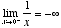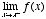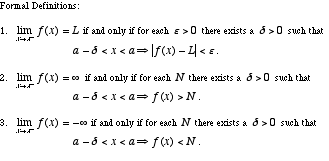index: click on a letter A B C D E F G H I J K L M N O P Q R S T U V W X Y Z A to Z index index: subject areas numbers & symbols sets, logic, proofs geometry algebra trigonometry advanced algebra & pre-calculus calculus advanced topics probability & statistics real world applications multimedia entrieswww.mathwords.com about mathwords website feedback

 Limit from the Left Limit from Below A one-sided limit which, in the example, restricts x such that x < 0. In general, a limit from the left restricts the domain variable to values less than the number the domain variable approaches. When a limit is taken from the left it is writtenor. For example,sincetends toward –∞ as x gets closer and closer to 0 from the left.See also

 this page updated 19-jul-17 Mathwords: Terms and Formulas from Algebra I to Calculus written, illustrated, and webmastered by Bruce Simmons Copyright © 2000 by Bruce Simmons All rights reserved